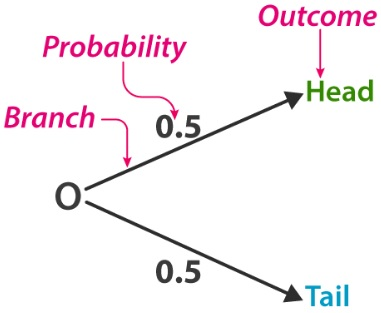Checkout JEE MAINS 2022 Question Paper Analysis : Checkout JEE MAINS 2022 Question Paper Analysis :

# Probability worksheets

Probability is the chance of some event to occur. When you need to know the probability of a particular event to take place, you think of the chances that you expect versus every possibility that can occur.

When you are not sure about the outcome of a particular event, you can specify the possibilities or how likely the outcome. Analysing the events that are governed by the probability is known as Statistics. Statistics Problems can be solved using Math formulas.A simple example that defines basics of probability is flipping a coin.

There are two possible outcomes after a coin is flipped, i.e,

• Tales

What is the probability of a fair coin (that has two different faces) that it lands on Heads?

Since there are only two possible outcomes that can occur out of one, hence the probability of the coin landing on Heads would be

Hence, P(H) = 0.5 or 50%

We bring you the Probability Math worksheets to help you improve in the probability concepts that include probability theory, probability statistics along with applications of probability.

## Probability Worksheet for Class 9

1. Two coins are tossed simultaneously 400 times and we get 2 heads equals to 180 times, one head = 148 times and no head = 70 times. If two coins are tossed at trandom, what is the probability of getting 2 heads , 1 head and 0 heads.
2. According to the meteorological report for 300 consecutive days in a year, its weather forecast was correct 180 times. Out of these days, one day was chosen at random, what is the probability that the weather forecast was correct on that day and not correct on that day.
3. In a cricket match, a batsman hit the boundary 5 times out of 40 balls played by him. Find the probability that the boundary is not hit by the ball.
4. In a survey of 200 ladies it was found that 142 like coffee, while 58 dislike it. Find the probability that a lady chosen at present likes coffee and dislike coffee.
5. In a cricket match, a batsman hits a boundary 6 times out of 30 balls he plays. Find the probability that he did not hit a ball.

Probability worksheet PDF for class 9 to solve more problems:-Download PDF Here

## Probability Worksheet for Class 10

1. A coin is tossed once, what is the probability of getting a head
2. A die is thrown once, find the probability of getting an even number and a multiple of 3,
3. Two dice are thrown at the same time, find the probability that the sum of two numbers appearing on the top of the dice is more than nine.
4. A bag contains 5 red balls and some blue balls.if the probability of drawing a blue ball from the bag is thrice that of a red ball, find the number of blue balls in the bag.
5. One card is drawn at random from a well shuffled deck of 52 cards. Find the probability that the card drawn is a king, a red 8, a spade, a red card, the six of the club and a face card.

Probability worksheet PDF for class 10 to solve more problems:-Download PDF Here